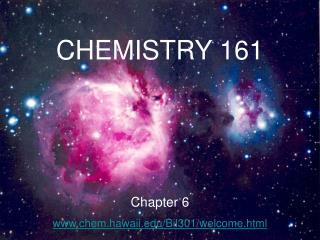DownloadDownload PresentationCHEMISTRY 161 Chapter 6 www.chem.hawaii.edu/Bil301/welcome.html

# CHEMISTRY 161 Chapter 6 www.chem.hawaii.edu/Bil301/welcome.html

Download Presentation## CHEMISTRY 161 Chapter 6 www.chem.hawaii.edu/Bil301/welcome.html

- - - - - - - - - - - - - - - - - - - - - - - - - - - E N D - - - - - - - - - - - - - - - - - - - - - - - - - - -
##### Presentation Transcript

1. CHEMISTRY 161 Chapter 6 www.chem.hawaii.edu/Bil301/welcome.html

2. THERMODYNAMICS HEAT CHANGE quantitative study of heat and energy changes of a system CH4(g) + 2 O2(g) → CO2(g) + 2 H2O(l) the state (condition) of a system is defined by T, p, n, V, E

3. the state (condition) of a system is defined by T, p, n, V, E STATE FUNCTIONS properties which depend only on the initial and final state, but not on the way how this condition was achieved

4. ΔV = Vfinal – Vinitial Δp = pfinal – pinitial ΔT = Tfinal – Tinitial ΔE = Efinal – Einitial

5. Energy is a STATE FUNCTION ΔE = m g Δh IT DOES NOT MATTER WHICH PATH YOU TAKE

6. Hess Law CH4(g) + 2O2(g) Reactants - 802 kJ - 890 kJ ENTHALPY, H CO2(g) + 2H2O(g) - 88 kJ CO2(g) + 2H2O(l) Products

7. Zeroth Law of Thermodynamics a system at thermodynamical equilibrium has a constant temperature heat is spontaneous transfer of thermal energy two bodies at different temperatures T1 > T2 spontaneous T2 T1

8. First Law of Thermodynamics energy can be converted from one form to another, but cannot be created or destroyed CONSERVATION OF ENERGY

9. SURROUNDINGS + - SYSTEM THE TOTAL ENERGY OF THE UNIVERSE IS CONSTANT ΔE = ΔEsystem + ΔEsurrounding = 0

10. First Law of Thermodynamics ΔEsystem = ΔQ + ΔW ΔQ heat change ΔW work done DQ > 0 ENDOTHERMIC ? DQ < 0 EXOTHERMIC

11. M M mechanical work ΔW = - p ΔV ΔV < 0 ΔV > 0 the energy of gas goes up the energy of gas goes down

12. First Law and Enthalpy ΔEsystem = ΔQ - pΔV • constant pressure → enthalpy change Δ H 2. ideal gas law → p V = n R T p ΔV = Δn R T ΔEsystem = ΔQ – R T Δn ΔQ = ΔEsystem + R T Δn = ΔH

13. ΔQ = ΔEsystem + R T Δn = ΔH Δ n = nfinal – ninitial Calculate the energy change of a system for the reaction process at 1 atm and 25C 2 CO(g) + O2(g) → 2 CO2(g) ΔHo = -566.0 kJ ΔEsystem = ΔH0 - R T Δn ΔEsystem = -563.5 kJ

14. A gas is compressed in a cylinder from a volume of 20 L to 2.0 L by a constant pressure of 10 atm. Calculate the amount of work done on the system.

15. Calculate the amount of work done against an atmospheric pressure of 1.00 atm when 500.0 g of zinc dissolves in excess acid at 30.0°C. Zn(s) + 2H+(aq) → Zn2+(aq) + H2 (g)

16. The heat of solution of KCl is +17.2 kJ/mol and the lattice energy of KCl(s) is 701.2 kJ/mol. Calculate the total heat of hydration of 1 mol of gas phase K+ ions and Cl– ions.

17. Homework Chapter 6, problems